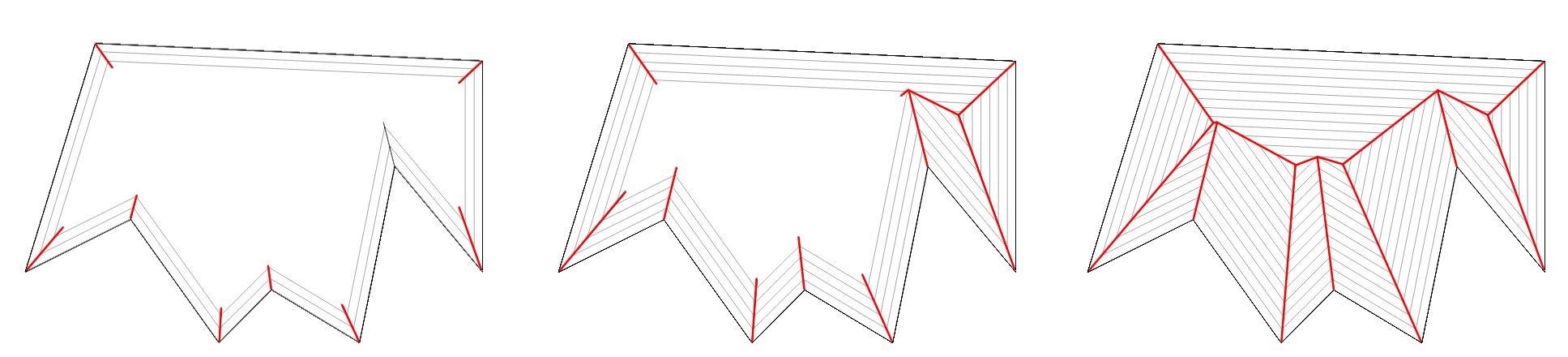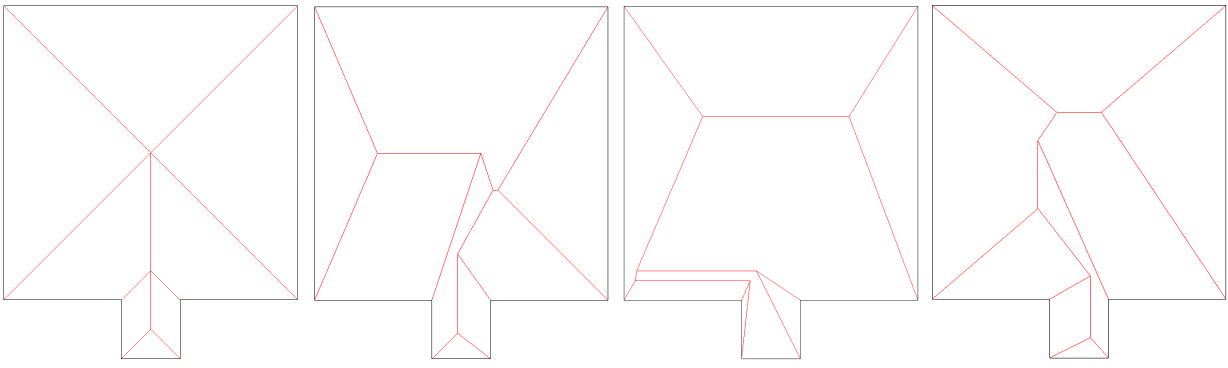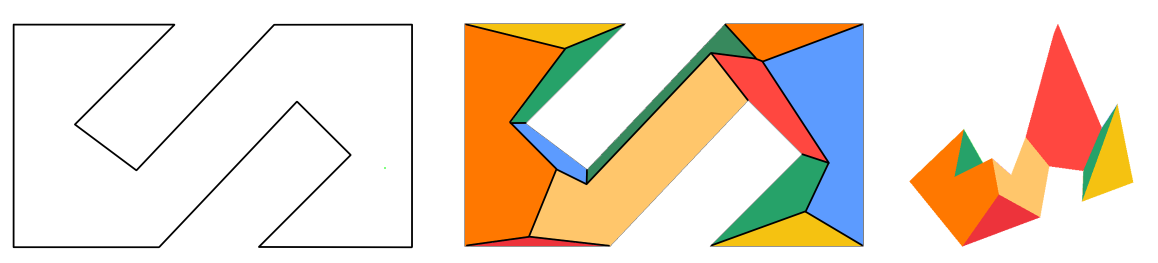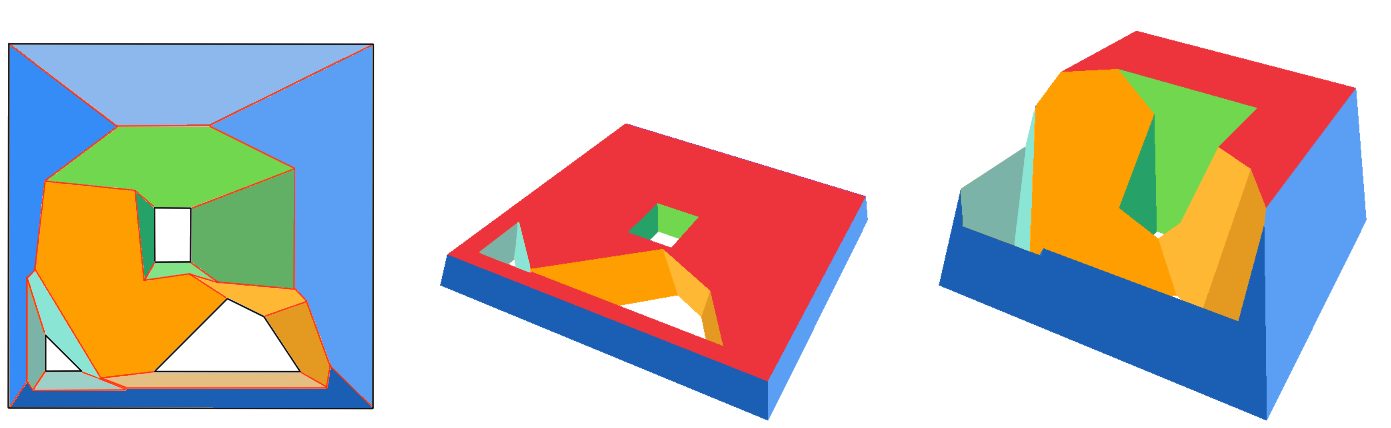# New in CGAL: Weighted Straight Skeletons and Straight Skeleton Extrusion

### Mael Rouxel-Labbé

#### GeometryFactory

Given a 2D polygon, an intuitive way to think of the construction of its straight skeleton is to imagine that wavefronts (or grassfires) are spawned at each edge of the polygon, and are moving inward. As the fronts progress, they either contract or expand depending on the angles formed between polygon edges, and sometimes disappear. Under this transformation, polygon vertices move along the angular bisector of the lines subtending the edges, tracing a tree-like structure: the straight skeleton.Construction of a straight skeleton: the wavefront interfaces define the straight skeleton (red).

Straight Skeletons are a fundamental tool in geometric modeling and computational geometry. They are used in a variety of applications, such as architecture, animation, and manufacturing. Straight Skeletons are also used as a building block for other algorithms, such as the offsetting of polygons, which is a fundamental operation in geometric modeling and computational geometry.

The straight skeleton of a polygon is similar to the medial axis and the Voronoi diagram of a polygon in the way it partitions it; however, unlike the medial axis and the Voronoi diagram, the bisectors are not equidistant to its defining edges but to the supporting lines of such edges. As a result, the bisectors of a straight skeleton might not be located in the center of the polygon and thus cannot be regarded as a proper medial axis in its geometrical meaning.

### Weighted Straight Skeletons

Weighted straight skeletons are a generalization of straight skeletons: contour edges are assigned a weight, which can be understood as assigning a speed to the wavefront spawned from the contour edge. Vertices no longer move along the angular bisector between two contour edges, but along a *weighted* bisector, which changes its angle.An unweighted straight skeleton (leftmost) and three randomly weighted straight skeletons.

The Straight Skeleton package of CGAL now supports the construction of weighted straight skeletons, with the restriction that all weights must be positive. For clarity, this functionality is implemented in a new set of functions: for existing functions such as `CGAL::create_..._straight_skeleton_2()`, there now exists a weighted equivalent: `CGAL::create_*weighted*_..._straight_skeleton_2()`.

### Straight Skeleton Extrusion

Perhaps the first (historically) use-case of straight skeletons was roof construction: given a polygon representing the boundary of the roof, the straight skeleton directly gives the layout of each tent. If each skeleton edge is lifted from the plane a height equal to its offset distance (also sometimes called "time"), the resulting roof is "correct" in that water will always fall down to the contour edges (the roof's border), regardless of where it falls on the roof.

The Straight Skeleton package of CGAL now implements skeleton extrusion for polygons with holes, with support for positive multiplicative weights. The output is a closed, combinatorially 2-manifold surface triangle mesh.Input polygon (left), weighted skeleton with colored faces (middle), and extruded skeleton (right).

Furthermore, it is possible to provide a maximum height, which will truncate the skeleton faces.Input polygon with three holes and its weighted straight skeleton (left),
and two extrusions of the skeleton with different maximum heights (middle and right).

### Updated User Manual

With the addition of these new features, the complete documentation of the package has been substancially updated. In particular, the user manual has been fully rewritten and extended.

### Status

All these new improvements are already integrated in CGAL's master branch on the CGAL GitHub repository, and will be officially released in the upcoming version of CGAL, CGAL 5.6, scheduled for June 2023.